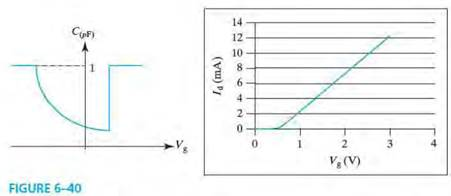# CV and Id - Vg characteristics of a hypothetical MOSFET with channel length L = I µm are given in Fig. 6-40. (a) Is the CV characteristic obtained at high frequency or low frequency? Or. is it impossible to determine? Explain. (b) Is this a PMOSFET or an NMOSFET? (c) Find the threshold voltage of this transistor. (d) Determine the mobility of the carriers in the channel of the transistor. (e) Plot Id - Vd curves at Vg = IV and Vg = 2.5 V.

Question
1 views

CV and Id - Vg characteristics of a hypothetical MOSFET with channel length L = I µm are given in Fig. 6-40.

(a) Is the CV characteristic obtained at high frequency or low frequency? Or. is it impossible to determine? Explain.

(b) Is this a PMOSFET or an NMOSFET?

(c) Find the threshold voltage of this transistor.

(d) Determine the mobility of the carriers in the channel of the transistor.

(e) Plot Id - Vd curves at Vg = IV and Vg = 2.5 V.### This question hasn't been answered yet.